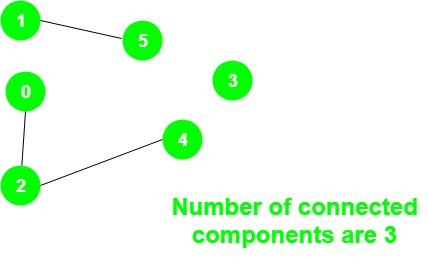Skip to content
Related Articles
Program to count Number of connected components in an undirected graph
• Difficulty Level : Easy
• Last Updated : 01 Dec, 2020

Given an undirected graph g, the task is to print the number of connected components in the graph.

Examples:

Input:Output:
There are three connected components:
1 – 5, 0 – 2 – 4 and 3

Approach: The idea is to use a variable count to store the number of connected components and do the following steps:

1. Initialize all vertices as unvisited.
2. For all the vertices check if a vertex has not been visited, then perform DFS on that vertex and increment the variable count by 1.

Below is the implementation of the above approach:

## C++

 `// C++ program for above approach``#include ``using` `namespace` `std;` `// Graph class represents a undirected graph``// using adjacency list representation``class` `Graph {``    ``// No. of vertices``    ``int` `V;` `    ``// Pointer to an array containing adjacency lists``    ``list<``int``>* adj;` `    ``// A function used by DFS``    ``void` `DFSUtil(``int` `v, ``bool` `visited[]);` `public``:``    ``// Constructor``    ``Graph(``int` `V);` `    ``void` `addEdge(``int` `v, ``int` `w);``    ``int` `NumberOfconnectedComponents();``};` `// Function to return the number of``// connected components in an undirected graph``int` `Graph::NumberOfconnectedComponents()``{` `    ``// Mark all the vertices as not visited``    ``bool``* visited = ``new` `bool``[V];` `    ``// To store the number of connected components``    ``int` `count = 0;``    ``for` `(``int` `v = 0; v < V; v++)``        ``visited[v] = ``false``;` `    ``for` `(``int` `v = 0; v < V; v++) {``        ``if` `(visited[v] == ``false``) {``            ``DFSUtil(v, visited);``            ``count += 1;``        ``}``    ``}` `    ``return` `count;``}` `void` `Graph::DFSUtil(``int` `v, ``bool` `visited[])``{` `    ``// Mark the current node as visited``    ``visited[v] = ``true``;` `    ``// Recur for all the vertices``    ``// adjacent to this vertex``    ``list<``int``>::iterator i;` `    ``for` `(i = adj[v].begin(); i != adj[v].end(); ++i)``        ``if` `(!visited[*i])``            ``DFSUtil(*i, visited);``}` `Graph::Graph(``int` `V)``{``    ``this``->V = V;``    ``adj = ``new` `list<``int``>[V];``}` `// Add an undirected edge``void` `Graph::addEdge(``int` `v, ``int` `w)``{``    ``adj[v].push_back(w);``    ``adj[w].push_back(v);``}` `// Driver code``int` `main()``{``    ``Graph g(5);``    ``g.addEdge(1, 0);``    ``g.addEdge(2, 3);``    ``g.addEdge(3, 4);` `    ``cout << g.NumberOfconnectedComponents();` `    ``return` `0;``}`

## Java

 `// Java program for above approach``import` `java.io.*;``import` `java.util.*;` `public` `class` `ConnectedComponentCount``{``    ` `    ``private` `int` `v;``    ``private` `int` `e;``    ``private` `Map> adjMap;``    ``private` `static` `Map visited;``    ` `    ``ConnectedComponentCount(``int` `vertices)``    ``{``        ``v = vertices;``        ``adjMap = ``new` `HashMap>();``        ``visited = ``new` `HashMap();``    ``}``    ` `    ``// Function to add edges``    ``private` `void` `addEdge(``int` `s, ``int` `d)``    ``{``        ``adjMap.putIfAbsent(s,``                     ``new` `HashSet());``        ``adjMap.putIfAbsent(d,``                     ``new` `HashSet());``        ``adjMap.get(s).add(d);``        ``adjMap.get(s).add(s);``        ``adjMap.get(d).add(s);``        ``adjMap.get(d).add(d);``        ``visited.put(s, ``0``);``        ``visited.put(d, ``0``);``    ``}``    ` `    ``// To mark vertices which can be visites``    ``private` `void` `findDFS(``int` `vertex)``    ``{``        ` `        ``// Mark as visited``        ``visited.put(vertex,``1``);``      ` `        ``// Print the vertex``        ``System.out.println(``"vertex "` `+ vertex +``                                   ``" visited"``);``        ``for``(Integer child : adjMap.get(vertex))``        ``{``            ``if``(visited.get(child) == ``0``){``               ``findDFS(child);``            ``}``        ``}``    ``}``    ` `    ``// Function to print graph``    ``private` `void` `printGraph()``    ``{``        ``for``(HashSet v :``                          ``adjMap.values())``        ``{``            ``System.out.println(v.toString());``        ``}``    ``}``    ` `    ``// Driver Code``    ``public` `static` `void` `main(String args[])``    ``{``        ` `        ``Scanner sc = ``new` `Scanner(System.in);``        ``System.out.println("Enter the number``                           ``of vertices (V): ");``        ``int` `vertices = sc.nextInt();``        ` `        ``System.out.println("Enter the number``                                ``of edges (E): ");``        ``int` `edges = sc.nextInt();``        ` `        ``// To count total number of``        ``// components``        ``int` `ccCount = ``0``;``        ` `        ``ConnectedComponentCount ccc =``                 ``new` `ConnectedComponentCount(``                                      ``vertices);``        ` `        ``// Input of edges``        ``while` `(edges > ``0``)``        ``{``            ``System.out.println("Enter the``                                ``nodes s & d: ");``            ``int` `s = sc.nextInt();``            ``int` `d = sc.nextInt();``            ``ccc.addEdge(s,d);``            ``edges-- ;``        ``}``        ` `        ``// Function to print graph                     ``        ``ccc.printGraph();``        ` `        ``// Traversing every node``        ``for``(Integer vertex : visited.keySet())``        ``{``            ` `            ``// Check if vertex is already``            ``// visited  or not``            ``if``(visited.get(vertex) == ``0``)``            ``{``                ` `                ``// Function Call for findDFS``                ``ccc.findDFS(vertex);``              ` `                ``// Print Component Found``                ``System.out.println(``"CC Found"``);``              ` `                ``// Increase the counter``                ``ccCount++;``            ``}``        ``}``        ` `        ``// Print number of components                       ``        ``System.out.println(``"Number of cc component: "``                                        ``+ ccCount);``    ``}``}` `//The code is contributed by Alfred Skaria`

## Python3

 `# Python3 program for above approach` `# Graph class represents a undirected graph``# using adjacency list representation``class` `Graph:``    ` `    ``def` `__init__(``self``, V):` `        ``# No. of vertices``        ``self``.V ``=` `V` `        ``# Pointer to an array containing``        ``# adjacency lists``        ``self``.adj ``=` `[[] ``for` `i ``in` `range``(``self``.V)]` `    ``# Function to return the number of``    ``# connected components in an undirected graph``    ``def` `NumberOfconnectedComponents(``self``):``        ` `        ``# Mark all the vertices as not visited``        ``visited ``=` `[``False` `for` `i ``in` `range``(``self``.V)]``        ` `        ``# To store the number of connected``        ``# components``        ``count ``=` `0``        ` `        ``for` `v ``in` `range``(``self``.V):``            ``if` `(visited[v] ``=``=` `False``):``                ``self``.DFSUtil(v, visited)``                ``count ``+``=` `1``                ` `        ``return` `count``        ` `    ``def` `DFSUtil(``self``, v, visited):` `        ``# Mark the current node as visited``        ``visited[v] ``=` `True` `        ``# Recur for all the vertices``        ``# adjacent to this vertex``        ``for` `i ``in` `self``.adj[v]:``            ``if` `(``not` `visited[i]):``                ``self``.DFSUtil(i, visited)``                ` `    ``# Add an undirected edge``    ``def` `addEdge(``self``, v, w):``        ` `        ``self``.adj[v].append(w)``        ``self``.adj[w].append(v)``        ` `# Driver code       ``if` `__name__``=``=``'__main__'``:``    ` `    ``g ``=` `Graph(``5``)``    ``g.addEdge(``1``, ``0``)``    ``g.addEdge(``2``, ``3``)``    ``g.addEdge(``3``, ``4``)``    ` `    ``print``(g.NumberOfconnectedComponents())` `# This code is contributed by rutvik_56`
Output
`2`

Attention reader! Don’t stop learning now. Get hold of all the important DSA concepts with the DSA Self Paced Course at a student-friendly price and become industry ready.  To complete your preparation from learning a language to DS Algo and many more,  please refer Complete Interview Preparation Course.

In case you wish to attend live classes with industry experts, please refer Geeks Classes Live

My Personal Notes arrow_drop_up